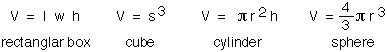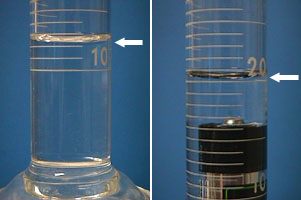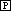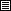Measuring Volume of Solids

## Measuring the Volume of Solids

The method for determining the volume of a solid depends on its shape.

Regular Geometric Shapes

The volume of a solid object with a regular geometric shape (rectangular box, cube, cylinder, sphere) can be determined using the volume formula for the shape.For example, a good approximation of the volume of the battery shown below could be made using the formula for the volume of a cylinder.This battery measured 4.94 cm in height and 1.42 cm in diameter, giving a volume of 7.82 cm3 or 7.82 ml.

Irregularly Shaped Objects

Because many objects are not regularly shaped their volume cannot be determined using a volume formula. The volume of these objects can be found by water displacement. A volume of water sufficient to cover the object is placed in a graduated cylinder and the volume read. The object is added to the cylinder and the volume read again. The difference between the two volumes is the volume of the object. This method is demonstrated using the same battery used above.The first volume reading (on the left) is 12.4 ml. After the battery has been added, the second volume reading (on the right) is 20.2 ml. The volume of the battery is 20.2 - 12.4 or 7.8 ml.